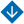State Estimation Methods for Fuzzy Integral Models. Part I: Approximation Methods
•About Advisory & Editorial Boards Aims & Scope Publication Ethics Review Process Archive Contact Information

#State Estimation Methods for Fuzzy Integral Models. Part I: Approximation Methods

Demenkov, N.P., Mikrin, E.A., and Mochalov, I.A. State Estimation Methods for Fuzzy Integral Models. Part I: Approximation Methods

Abstract. The existing and newly proposed methods for estimating the state of integral models with fuzzy uncertainty are reviewed. A fuzzy integral model with the limit transition defined in the Hausdorff metric is introduced. This model is used to formulate the state estimation problem for the models described by fuzzy Fredholm–Volterra integral equations. Several fuzzy methods for solving this problem are considered as follows: the fuzzy Laplace transform, the method of “embedding” models (transforming an original system into a higher dimension system and solving the resulting problem by traditional linear algebra methods), the Taylor estimation of the degenerate nuclei under the integral sign that are represented by power polynomials, and the estimation of the nondegenerate nuclei by degenerate forms using the Taylor approximation. As shown below, in some cases, the estimation results are related to the solution of fuzzy systems of linear algebraic equations. Test examples are solved for them.

Keywords: fuzzy Riemann integral, fuzzy integral model, fuzzy methods for estimating integral models.

PDF (English)